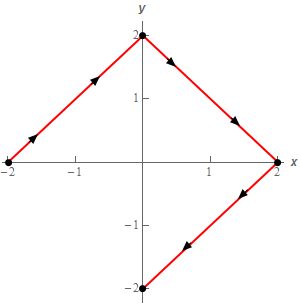Paul's Online Notes
Home / Calculus III / Line Integrals / Fundamental Theorem for Line Integrals
Show Mobile Notice Show All Notes Hide All Notes
Mobile Notice
You appear to be on a device with a "narrow" screen width (i.e. you are probably on a mobile phone). Due to the nature of the mathematics on this site it is best views in landscape mode. If your device is not in landscape mode many of the equations will run off the side of your device (should be able to scroll to see them) and some of the menu items will be cut off due to the narrow screen width.

### Section 16.5 : Fundamental Theorem for Line Integrals

4. Evaluate $$\displaystyle \int\limits_{C}{{\nabla f\centerdot d\vec r}}$$ where $$f\left( {x,y} \right) = {{\bf{e}}^{x\,y}} - {x^2} + {y^3}$$ and $$C$$ is the curve shown below.Show Solution

This problem is much simpler than it appears at first. We do not need to compute 3 different line integrals (one for each curve in the sketch).

All we need to do is notice that we are doing a line integral for a gradient vector function and so we can use the Fundamental Theorem for Line Integrals to do this problem.

Using the Fundamental Theorem to evaluate the integral gives the following,

\begin{align*}\int\limits_{C}{{\nabla f\centerdot d\vec r}} &= f\left( {{\rm{end point}}} \right) - f\left( {{\rm{start point}}} \right)\\ & = f\left( {0, - 2} \right) - f\left( { - 2,0} \right)\\ & = - 7 - \left( { - 3} \right) = \require{bbox} \bbox[2pt,border:1px solid black]{{ - 4}}\end{align*}

Remember that all the Fundamental Theorem requires is the starting and ending point of the curve and the function used to generate the gradient vector field.